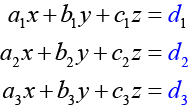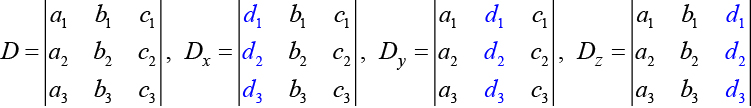# 7.8 Solving systems with cramer's rule  (Page 3/11)

 Page 3 / 11The algebra is as follows:

$|A|={a}_{1}{b}_{2}{c}_{3}+{b}_{1}{c}_{2}{a}_{3}+{c}_{1}{a}_{2}{b}_{3}-{a}_{3}{b}_{2}{c}_{1}-{b}_{3}{c}_{2}{a}_{1}-{c}_{3}{a}_{2}{b}_{1}$

## Finding the determinant of a 3 × 3 matrix

Find the determinant of the 3 × 3 matrix given

$A=\left[\begin{array}{ccc}0& 2& 1\\ 3& -1& 1\\ 4& 0& 1\end{array}\right]$

Augment the matrix with the first two columns and then follow the formula. Thus,

$\begin{array}{l}|A|=|\begin{array}{ccc}0& 2& 1\\ 3& -1& 1\\ 4& 0& 1\end{array}\text{\hspace{0.17em}}\text{\hspace{0.17em}}|\begin{array}{c}0\\ 3\\ \text{\hspace{0.17em}}\text{\hspace{0.17em}}4\end{array}\text{\hspace{0.17em}}\text{\hspace{0.17em}}\text{\hspace{0.17em}}\text{\hspace{0.17em}}\begin{array}{c}2\\ -1\\ 0\end{array}|\hfill \\ \text{\hspace{0.17em}}\text{\hspace{0.17em}}\text{\hspace{0.17em}}\text{\hspace{0.17em}}\text{\hspace{0.17em}}\text{\hspace{0.17em}}\text{\hspace{0.17em}}=0\left(-1\right)\left(1\right)+2\left(1\right)\left(4\right)+1\left(3\right)\left(0\right)-4\left(-1\right)\left(1\right)-0\left(1\right)\left(0\right)-1\left(3\right)\left(2\right)\hfill \\ \text{\hspace{0.17em}}\text{\hspace{0.17em}}\text{\hspace{0.17em}}\text{\hspace{0.17em}}\text{\hspace{0.17em}}\text{\hspace{0.17em}}\text{\hspace{0.17em}}=0+8+0+4-0-6\hfill \\ \text{\hspace{0.17em}}\text{\hspace{0.17em}}\text{\hspace{0.17em}}\text{\hspace{0.17em}}\text{\hspace{0.17em}}\text{\hspace{0.17em}}\text{\hspace{0.17em}}=6\hfill \end{array}$

Find the determinant of the 3 × 3 matrix.

$\mathrm{det}\left(A\right)=|\begin{array}{ccc}1& -3& 7\\ 1& 1& 1\\ 1& -2& 3\end{array}|$

$-10$

Can we use the same method to find the determinant of a larger matrix?

No, this method only works for and matrices. For larger matrices it is best to use a graphing utility or computer software.

## Using cramer’s rule to solve a system of three equations in three variables

Now that we can find the determinant    of a 3 × 3 matrix, we can apply Cramer’s Rule    to solve a system of three equations in three variables . Cramer’s Rule is straightforward, following a pattern consistent with Cramer’s Rule for 2 × 2 matrices. As the order of the matrix increases to 3 × 3, however, there are many more calculations required.

When we calculate the determinant to be zero, Cramer’s Rule gives no indication as to whether the system has no solution or an infinite number of solutions. To find out, we have to perform elimination on the system.

Consider a 3 × 3 system of equations.$x=\frac{{D}_{x}}{D},y=\frac{{D}_{y}}{D},z=\frac{{D}_{z}}{D},D\ne 0$

whereIf we are writing the determinant $\text{\hspace{0.17em}}{D}_{x},$ we replace the $\text{\hspace{0.17em}}x\text{\hspace{0.17em}}$ column with the constant column. If we are writing the determinant ${D}_{y},$ we replace the $\text{\hspace{0.17em}}y\text{\hspace{0.17em}}$ column with the constant column. If we are writing the determinant $\text{\hspace{0.17em}}{D}_{z},$ we replace the $\text{\hspace{0.17em}}z\text{\hspace{0.17em}}$ column with the constant column. Always check the answer.

## Solving a 3 × 3 system using cramer’s rule

Find the solution to the given 3 × 3 system using Cramer’s Rule.

$\begin{array}{c}x+y-z=6\\ 3x-2y+z=-5\\ x+3y-2z=14\end{array}$

Use Cramer’s Rule.

$D=|\begin{array}{ccc}1& \text{\hspace{0.17em}}\text{\hspace{0.17em}}1& -1\\ 3& -2& \text{\hspace{0.17em}}\text{\hspace{0.17em}}\text{\hspace{0.17em}}1\\ 1& \text{\hspace{0.17em}}\text{\hspace{0.17em}}3& -2\end{array}|,{D}_{x}=|\begin{array}{ccc}6& 1& -1\\ -5& -2& \text{\hspace{0.17em}}\text{\hspace{0.17em}}\text{\hspace{0.17em}}1\\ 14& \text{\hspace{0.17em}}\text{\hspace{0.17em}}3& -2\end{array}|,{D}_{y}=|\begin{array}{ccc}1& \text{\hspace{0.17em}}6& -1\\ 3& -5& \text{\hspace{0.17em}}\text{\hspace{0.17em}}1\\ 1& 14& -2\end{array}|,{D}_{z}=|\begin{array}{ccc}1& \text{\hspace{0.17em}}1& 6\\ 3& -2& -5\\ 1& \text{\hspace{0.17em}}\text{\hspace{0.17em}}3& 14\end{array}|$

Then,

$\begin{array}{l}x=\frac{{D}_{x}}{D}=\frac{-3}{-3}=1\hfill \\ y=\frac{{D}_{y}}{D}=\frac{-9}{-3}=3\hfill \\ z=\frac{{D}_{z}}{D}=\frac{6}{-3}=-2\hfill \end{array}$

The solution is $\left(1,3,-2\right).$

Use Cramer’s Rule to solve the 3 × 3 matrix.

$\begin{array}{r}\hfill x-3y+7z=13\\ \hfill x+y+z=1\text{\hspace{0.17em}}\text{\hspace{0.17em}}\text{\hspace{0.17em}}\\ \hfill x-2y+3z=4\text{\hspace{0.17em}}\text{\hspace{0.17em}}\text{\hspace{0.17em}}\end{array}$

$\left(-2,\frac{3}{5},\frac{12}{5}\right)$

## Using cramer’s rule to solve an inconsistent system

Solve the system of equations using Cramer’s Rule.

$\begin{array}{l}3x-2y=4\text{ }\left(1\right)\\ 6x-4y=0\text{ }\left(2\right)\end{array}$

We begin by finding the determinants

$D=|\begin{array}{cc}3& -2\\ 6& -4\end{array}|=3\left(-4\right)-6\left(-2\right)=0$

We know that a determinant of zero means that either the system has no solution or it has an infinite number of solutions. To see which one, we use the process of elimination. Our goal is to eliminate one of the variables.

1. Multiply equation (1) by $\text{\hspace{0.17em}}-2.$
2. Add the result to equation $\text{\hspace{0.17em}}\left(2\right).$
$\begin{array}{l}\underset{_______________}{\begin{array}{l}\begin{array}{l}\hfill \\ -6x+4y\text{\hspace{0.17em}}\text{\hspace{0.17em}}\text{\hspace{0.17em}}\text{\hspace{0.17em}}=-8\hfill \end{array}\hfill \\ \text{\hspace{0.17em}}\text{\hspace{0.17em}}\text{\hspace{0.17em}}6x-4y\text{\hspace{0.17em}}\text{\hspace{0.17em}}\text{\hspace{0.17em}}\text{\hspace{0.17em}}\text{\hspace{0.17em}}\text{\hspace{0.17em}}=\text{\hspace{0.17em}}\text{\hspace{0.17em}}\text{\hspace{0.17em}}\text{\hspace{0.17em}}0\hfill \end{array}}\hfill \\ \text{\hspace{0.17em}}\text{\hspace{0.17em}}\text{\hspace{0.17em}}\text{\hspace{0.17em}}\text{\hspace{0.17em}}\text{\hspace{0.17em}}\text{\hspace{0.17em}}\text{\hspace{0.17em}}\text{\hspace{0.17em}}\text{\hspace{0.17em}}\text{\hspace{0.17em}}\text{\hspace{0.17em}}\text{\hspace{0.17em}}\text{\hspace{0.17em}}\text{\hspace{0.17em}}\text{\hspace{0.17em}}\text{\hspace{0.17em}}\text{\hspace{0.17em}}\text{\hspace{0.17em}}0\text{\hspace{0.17em}}\text{\hspace{0.17em}}\text{\hspace{0.17em}}\text{\hspace{0.17em}}\text{\hspace{0.17em}}\text{\hspace{0.17em}}=\text{\hspace{0.17em}}-8\hfill \end{array}$

We obtain the equation $\text{\hspace{0.17em}}0=-8,\text{\hspace{0.17em}}$ which is false. Therefore, the system has no solution. Graphing the system reveals two parallel lines. See [link] .

## Use cramer’s rule to solve a dependent system

Solve the system with an infinite number of solutions.

$\begin{array}{rr}\hfill x-2y+3z=0& \hfill \left(1\right)\\ \hfill 3x+y-2z=0& \hfill \left(2\right)\\ \hfill 2x-4y+6z=0& \hfill \left(3\right)\end{array}$

Let’s find the determinant first. Set up a matrix augmented by the first two columns.

Then,

$1\left(1\right)\left(6\right)+\left(-2\right)\left(-2\right)\left(2\right)+3\left(3\right)\left(-4\right)-2\left(1\right)\left(3\right)-\left(-4\right)\left(-2\right)\left(1\right)-6\left(3\right)\left(-2\right)=0$

As the determinant equals zero, there is either no solution or an infinite number of solutions. We have to perform elimination to find out.

1. Multiply equation (1) by $\text{\hspace{0.17em}}-2\text{\hspace{0.17em}}$ and add the result to equation (3):
$\frac{\begin{array}{r}\hfill -2x+4y-6x=0\\ \hfill 2x-4y+6z=0\end{array}}{\text{\hspace{0.17em}}\text{\hspace{0.17em}}\text{\hspace{0.17em}}\text{\hspace{0.17em}}\text{\hspace{0.17em}}\text{\hspace{0.17em}}\text{\hspace{0.17em}}\text{\hspace{0.17em}}\text{\hspace{0.17em}}\text{\hspace{0.17em}}\text{\hspace{0.17em}}\text{\hspace{0.17em}}\text{\hspace{0.17em}}\text{\hspace{0.17em}}\text{\hspace{0.17em}}\text{\hspace{0.17em}}\text{\hspace{0.17em}}\text{\hspace{0.17em}}\text{\hspace{0.17em}}\text{\hspace{0.17em}}\text{\hspace{0.17em}}\text{\hspace{0.17em}}\text{\hspace{0.17em}}\text{\hspace{0.17em}}\text{\hspace{0.17em}}\text{\hspace{0.17em}}\text{\hspace{0.17em}}\text{\hspace{0.17em}}\text{\hspace{0.17em}}\text{\hspace{0.17em}}\text{\hspace{0.17em}}0=0}$
2. Obtaining an answer of $\text{\hspace{0.17em}}0=0,\text{\hspace{0.17em}}$ a statement that is always true, means that the system has an infinite number of solutions. Graphing the system, we can see that two of the planes are the same and they both intersect the third plane on a line. See [link] .

explain and give four Example hyperbolic function
The denominator of a certain fraction is 9 more than the numerator. If 6 is added to both terms of the fraction, the value of the fraction becomes 2/3. Find the original fraction. 2. The sum of the least and greatest of 3 consecutive integers is 60. What are the valu
1. x + 6 2 -------------- = _ x + 9 + 6 3 x + 6 3 ----------- x -- (cross multiply) x + 15 2 3(x + 6) = 2(x + 15) 3x + 18 = 2x + 30 (-2x from both) x + 18 = 30 (-18 from both) x = 12 Test: 12 + 6 18 2 -------------- = --- = --- 12 + 9 + 6 27 3
Pawel
2. (x) + (x + 2) = 60 2x + 2 = 60 2x = 58 x = 29 29, 30, & 31
Pawel
ok
Ifeanyi
on number 2 question How did you got 2x +2
Ifeanyi
combine like terms. x + x + 2 is same as 2x + 2
Pawel
Mark and Don are planning to sell each of their marble collections at a garage sale. If Don has 1 more than 3 times the number of marbles Mark has, how many does each boy have to sell if the total number of marbles is 113?
Mark = x,. Don = 3x + 1 x + 3x + 1 = 113 4x = 112, x = 28 Mark = 28, Don = 85, 28 + 85 = 113
Pawel
how do I set up the problem?
what is a solution set?
Harshika
find the subring of gaussian integers?
Rofiqul
hello, I am happy to help!
Abdullahi
hi mam
Mark
find the value of 2x=32
divide by 2 on each side of the equal sign to solve for x
corri
X=16
Michael
Want to review on complex number 1.What are complex number 2.How to solve complex number problems.
Beyan
yes i wantt to review
Mark
use the y -intercept and slope to sketch the graph of the equation y=6x
how do we prove the quadratic formular
Darius
hello, if you have a question about Algebra 2. I may be able to help. I am an Algebra 2 Teacher
thank you help me with how to prove the quadratic equation
Seidu
may God blessed u for that. Please I want u to help me in sets.
Opoku
what is math number
4
Trista
x-2y+3z=-3 2x-y+z=7 -x+3y-z=6
can you teacch how to solve that🙏
Mark
Solve for the first variable in one of the equations, then substitute the result into the other equation. Point For: (6111,4111,−411)(6111,4111,-411) Equation Form: x=6111,y=4111,z=−411x=6111,y=4111,z=-411
Brenna
(61/11,41/11,−4/11)
Brenna
x=61/11 y=41/11 z=−4/11 x=61/11 y=41/11 z=-4/11
Brenna
Need help solving this problem (2/7)^-2
x+2y-z=7
Sidiki
what is the coefficient of -4×
-1
Shedrak
the operation * is x * y =x + y/ 1+(x × y) show if the operation is commutative if x × y is not equal to -1ByByBy John GabrieliBy Vanessa SoledadBy Qqq QqqBy Brooke DelaneyBy Laurence BailenBy Rylee MinllicBy RhodesBy Caitlyn GobbleBy Robert MurphyBy OpenStax